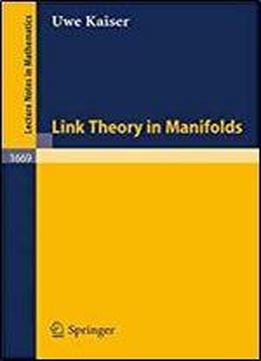Link Theory In Manifolds (lecture Notes In Mathematics, Vol. 1669) by Uwe Kaiser / 1997 / English / PDF

Any topological theory of knots and links should be based on simple ideas of intersection and linking. In this book, a general theory of link bordism in manifolds and universal constructions of linking numbers in oriented 3-manifolds are developed. In this way, classical concepts of link theory in the 3-spheres are generalized to a certain class of oriented 3-manifolds (submanifolds of rational homology 3-spheres). The techniques needed are described in the book but basic knowledge in topology and algebra is assumed. The book should be of interst to those working in topology, in particular knot theory and low-dimensional topology.

views: 351